Go vs Swift vs C++ 简单微型基准比较测试

【推荐阅读】滴滴出行的数据架构和信息流处理思路解析->>>Go

package main
import "fmt"

func main() {
var sum int64 = 0

for e := 0; e < 200; e++ {
sum = 0

var x[]int64
for i := 0 ; i < 1000000; i++ {
x = append(x, int64(i));
}

var y[]int64
for i := 0 ; i < 1000000-1; i++ {
y = append(y, x[i]+x[i+1]);
}

for i := 0 ; i < 1000000; i += 100 {
sum += y[i];
}

}

fmt.Println(sum);
}

Swift

var sum : Int64 = 0

for e in 0..<200 {
sum = 0

var x : [Int64] = []
for (var i = 0; i < 1000000; i++) {
x.append(Int64(i));
}

var y: [Int64] = []
for (var i = 0; i < 1000000-1; i++) {
y.append(x[i] + x[i+1]);
}

for (var i = 0; i < 1000000; i+=100) {
sum += y[i]
}
}

print(sum)

C++

#include <vector>
#include <iostream>

int main() {
int64_t sum = 0;

for(int e = 0; e < 200; e++) {
sum = 0;

std::vector<int64_t> x;
for(int i = 0; i < 1000000; i++) {
x.push_back(i);
}

std::vector<int64_t> y;
for(int i = 0; i < 1000000-1; i++) {
y.push_back(x[i] + x[i+1]);
}

for (int i = 0; i < 1000000; i += 100) {
sum += y[i];
}
}

std::cout << sum << std::endl;
}

go build -o test-go go/test.go
swiftc -O -o test-swift swift/test.swift
c++ -O3 -o test-c cplusplus/test.cc
GOMAXPROCS=2 time ./test-go
9999010000 4.32 real 5.99 user         0.38 sys
GOMAXPROCS=1 time ./test-go
9999010000 3.93 real 3.89 user         0.05 sys
time ./test-swift
9999010000 3.96 real 2.82 user         1.12 sys
time ./test-c
9999010000 2.37 real 1.74 user         0.62 sys

go build -o test-go go/test.go
swiftc -O -o test-swift swift/test.swift
c++ -O3 -o test-c cplusplus/test.cc
GOMAXPROCS=2 time ./test-go
99901000 4.40 real 5.65 user         1.10 sys
GOMAXPROCS=1 time ./test-go
99901000 5.06 real 5.07 user         0.83 sys
time ./test-swift
99901000 2.32 real 2.29 user         0.01 sys
time ./test-c
99901000 1.39 real 1.37 user         0.01 sys

1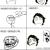C++ 用法不对哦楼主

#include <vector>
#include <iostream>

intmain() {
int64_t sum = 0;

for(inte = 0; e < 200; e++) {
sum = 0;

std::vector<int64_t> x;

x.resize(1000000);

for(inti = 0; i < 1000000; i++) {
x.push_back(i);
}

std::vector<int64_t> y;

y.resize(1000000);

for(inti = 0; i < 1000000-1; i++) {
y.push_back(x[i] + x[i+1]);
}

for(inti = 0; i < 1000000; i += 100) {
sum += y[i];
}
}

std::cout << sum << std::endl;

}0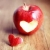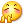沙发
0
c
go这么测试并不公平吧
0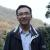var x = make([]int64, 0, 1000000)

var y = make([]int64, 0, 1000000)

Go的代码改下这个

0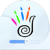0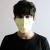0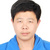围观!
0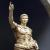swift不错， 而且有应用场景， 可以在IOS上做APP开发。

0
s